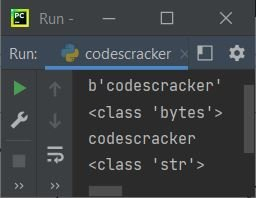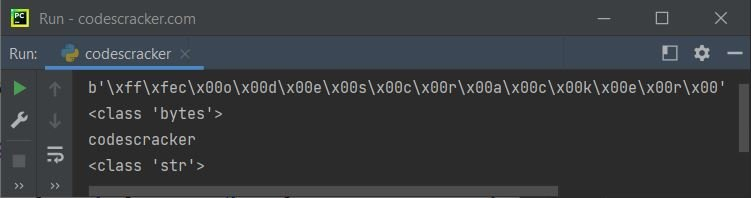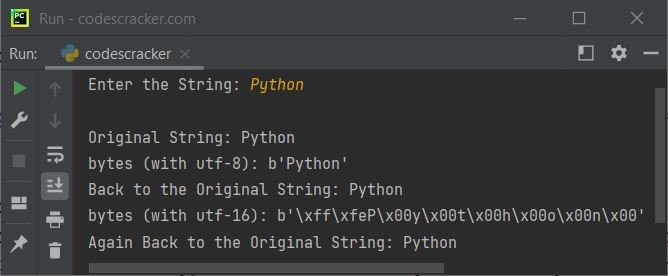# Python Program to Convert Bytes to a String

This article is created to cover a program in Python that converts bytes to a string. The question is: write a Python program to convert bytes to strings. The answer to this question is the program given below.

The program given below defines a bytes object named x and then prints the value and its type. Then it uses the decode() method to convert the bytes object to a string object, and then prints the value and type of the same variable again:

```x = b'codescracker'
print(x)
print(type(x))

x = x.decode()
print(x)
print(type(x))```

The snapshot given below shows the sample output produced by the above Python program:But the problem is that when the bytes do not contain UTF-8-encoded data, you need to specify the same decoding method that was used to encode the data. For example, in the following program, the variable x contains the bytes object encoded with UTF-16; therefore, while decoding into a string, we need to use the same UTF-16 decoding:

```x = b'\xff\xfec\x00o\x00d\x00e\x00s\x00c\x00r\x00a\x00c\x00k\x00e\x00r\x00'
print(x)
print(type(x))

x = x.decode("UTF-16")
print(x)
print(type(x))```

Here is its sample output:## bytes to a string in Python

Here is the last program in this article, created to allow the user to enter the string. The string gets converted to bytes with utf-8 encoding, and then bytes get converted to string again. Same thing done using utf-16 encoding and decoding:

```print("Enter the String: ", end="")
x = input()

print("\nOriginal String:", x)
x = bytes(x, "utf-8")
print("bytes (with utf-8):", x)
x = x.decode("utf-8")
print("Back to the Original String:", x)
x = bytes(x, "utf-16")
print("bytes (with utf-16):", x)
x = x.decode("utf-16")
print("Again Back to the Original String:", x)```

The sample run of the above program with user input "Python" is shown in the snapshot given below:Python Online Test

« Previous Program Next Program »